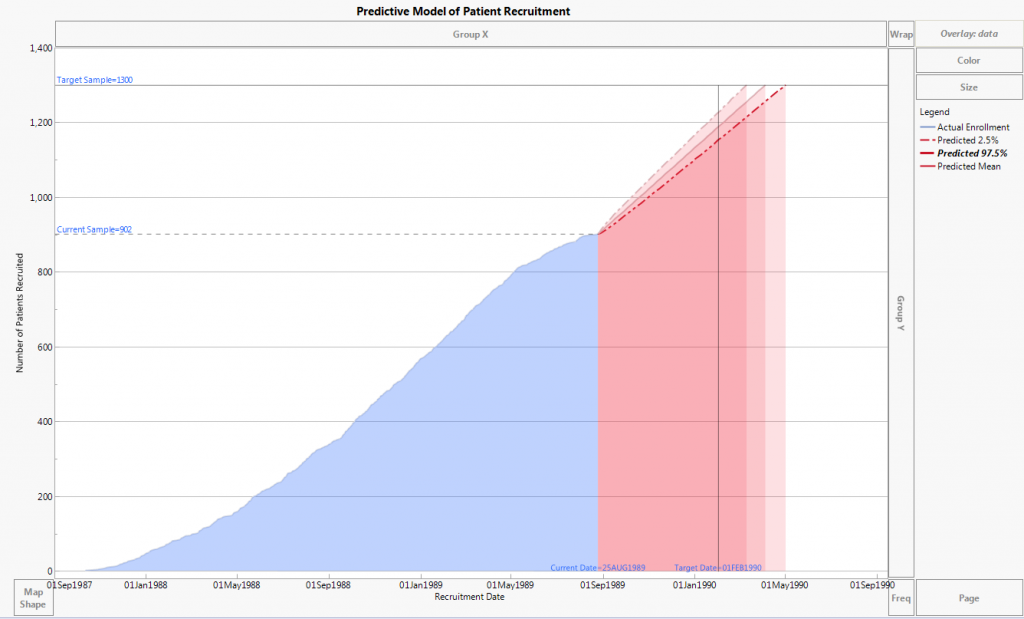The World Statistics Day celebration continues here in the Community. We all need reliable data for sound decision making. Do you have a data source that you trust most? Head over to Discussions to tell us about it.
Choose Language Hide Translation Bar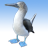phoebe_jiang
Staff (Retired)
Using data points to fill a graphic with color

When dealing with graphs and plots, we will very likely need to fill colors in a graph to highlight an area or distinguish it from other shapes. You may know how to shade regular shapes, but what about irregular polygons and contours? You can do can this easily to any graphical shapes using Polygon(). We just need the data points!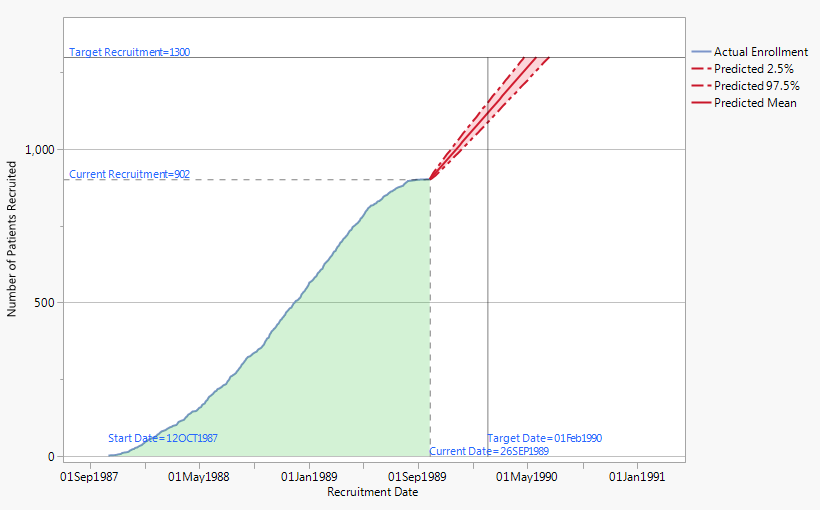Above is a graph I was working on for patient recruitment prediction (Look for a blog post about this soon). The dash-dot lines are 2.5th and 97.5th percentiles of 10,000 simulations. The solid red line is the mean of the simulated values. Filling this confidence interval with color would help people understand the scope of the enrollment prediction, so I explored how to do that. In the beginning, I specified the three vertices of the triangle and got this: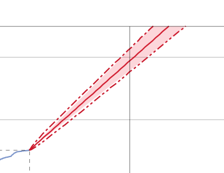Hmm…not quite what I expected. What’s the problem? Apparently this graphic is not exactly a triangle, although it sure looks like one. The bottom part is wider, and therefore the triangular shading could not cover the entire confidence interval band. How can we fix this?

To fill a graphic with color, you first need to know the boundary of your shape and the data points that make up the boundary. In this case, my boundaries are three sides of the “triangle,” and the data points are the 2.5th percentiles and 97.5th percentiles. Note that I do not need the third side, the horizontal line segment, because once I construct the two sides, JMP automatically connects the two end points with a straight line. But if you have a segment that's not straight, you would need data points to tell JMP where the shading stops.

Next, in JSL, we pull out the data points from the data set, save the data in columns and sort them.

`ds = Data Table( "graphbuilder" );`

ds << select where( :data == "Predicted 2.5%" );

dslow = ds << subset(

Output Table Name( "Lower Percentile" ),

Selected Rows( 1 ),

invisible

);

dslow_sort = dslow << sort( By( :time ), Order( Ascending ) );

ds << select where( :data == "Predicted 97.5%" );

dsupp = ds << subset(

Output Table Name( "Upper Percentile" ),

Selected Rows( 1 ),

invisible

);

dsupp_sort = dsupp << sort( By( :time ), Order( Descending ) );

The two subsets, dslow and dsupp, contain data points of our boundary, the two dash-dot lines. Note that I sorted dslow by ascending order but dsupp by descending order since JMP draws the boundary with direction. I chose to draw the CI band clockwise: starting from the intersection point of the blue line and the red lines, going up following the 2.5th percentile, and coming back following the 97.5th percentile from the horizontal side. Thus, the data points of the 97.5th percentile need to be in descending order.

Now that we’ve got the data points sorted, we need to put them together in a matrix named “all”. The x values in the matrix is “xall” and y values in the matrix is “yall”. Here, time is my x-axis and number is my y-axis.

`Col1 = Column( dslow_sort, "time" );`

tl = col << GetAsMatrix;

Col1 = Column( dslow_sort, "number" );

nl = col << GetAsMatrix;

Col2 = Column( dsupp_sort, "time" );

tu = col << GetAsMatrix;

col2 = Column( dsupp_sort, "number" );

nu = col << GetAsMatrix;

lower = tl || nl;

upper = tu || nu;

all = lower |/ upper;

xall = all[0,1];

yall = all[0,2];

`Pen Color( "red" );`

Fill Color( "red" );

Transparency( 0.2 );

Polygon(xall, yall);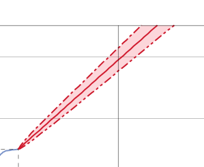The final step is easy: Call the matrix in Polygon() and fill in color. Use the code from the second box above in your Graph Builder statement or in a new window. You should now have a triangle band perfectly shaded in red with some degree of transparency!

Final thoughts: You can fill any shape of area with color as long as you have the data points. Below is another example of graphic script shading, which demonstrates just one of the many variations you can create by changing the options.